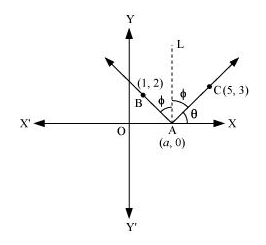Deepak Scored 45->99%ile with Bounce Back Crack Course. You can do it too!

# A ray of light passing through the point (1, 2) reflects on the x-axis

Question:

A ray of light passing through the point (1, 2) reflects on the x-axis at point A and the reflected ray passes through the point (5, 3). Find the coordinates of A.

Solution:Let the coordinates of point A be (a, 0).

Draw a line (AL) perpendicular to the x-axis.

We know that angle of incidence is equal to angle of reflection. Hence, let

$\angle B A L=\angle C A L=\Phi$

Let $\angle \mathrm{CAX}=\theta$

$\therefore \angle \mathrm{OAB}=180^{\circ}-(\theta+2 \Phi)=180^{\circ}-\left[\theta+2\left(90^{\circ}-\theta\right)\right]$

$=180^{\circ}-\theta-180^{\circ}+2 \theta$

$=\theta$

$\therefore \angle B A X=180^{\circ}-\theta$

Now, slope of line $\mathrm{AC}=\frac{3-0}{5-a}$

$\Rightarrow \tan \theta=\frac{3}{5-a}$ $\ldots(1)$

Slope of line $\mathrm{AB}=\frac{2-0}{1-a}$

$\Rightarrow \tan \left(180^{\circ}-\theta\right)=\frac{2}{1-a}$

$\Rightarrow-\tan \theta=\frac{2}{1-a}$

$\Rightarrow \tan \theta=\frac{2}{a-1}$ $\cdots(2)$

From equations (1) and (2), we obtain

$\frac{3}{5-a}=\frac{2}{a-1}$

$\Rightarrow 3 a-3=10-2 a$

$\Rightarrow a=\frac{13}{5}$

Thus, the coordinates of point $\mathrm{A}$ are $\left(\frac{13}{5}, 0\right)$.